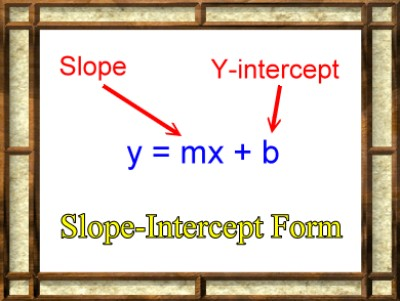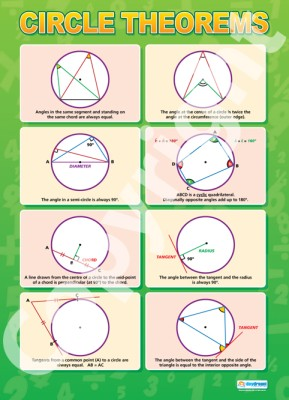List of public pages created with Protopage

# Unit A

## Plain sticky notes

### Unit A

Prime numbers Multiples, Factors and Prime Numbers LCM and HCF Rounding Numbers Ratios Metric and Imperial Units Sequences Finding the nth term Making Formulas from words Basic Algebra Solving Equations Rearranging Formulas Drawing Triangles Loci and Construction Pythagoras theorem and bearings Trigonometry 3D Pythagoras and trigonometry Coordinates Sampling Methods Mean, Median, Mode and Range

### Multiples, Factors and Prime Numbers

FACTORS The factors of a number are all the numbers which go into the number exactly, with no remainder. For example the factors of 6 are 1, 2, 3 and 6. Remember that 1 and the number itself are always factors of any number, and these two factors are called trivial factors because they are obvious. PRIME NUMBERS A prime number has no factors except 1 and itself, for example, 2, 3, 5 and 7 are all prime numbers. A prime number must have two factors, 1 and itself, so 1 is not a prime number. The prime numbers less than 60 are 2, 3, 5, 7, 11, 13, 17, 19, 23, 29, 31, 37, 41, 43, 47, 53 and 59 - it is helpful to be able to recognise them. MULTIPLES A multiple of a number is any number that it goes into, for example 6, 12, 18, 24, 30 and 36 are all multiples of 6. Any number is of course a multiple of itself since it goes into itself once. The multiples of a number are therefore the numbers that make up its “times table”.

### Prime Numbers

1) Prime Numbers don't divide by anthing. For example: The only numbers that multiply to give 7 are 1 x 7 The only numbers that multiply to give 31 are 1 x 31 The only way to get any prime number is 1 x itself. 2) They end in 1,3,7 or 9. 1) 1 is not a prime number. 2) The first four prime numbers are 2, 3, 5 and 7. 3) Prime numbers end in 1, 3, 7 or 9. (2 and 5 are the only exception for this rule.) 4) But not all numbers ending in 1, 3, 7 or 9 are primes.

### LMC and HCF

HCF The highest common factor (HCF) of two whole numbers is the largest whole number which is a factor of both. Consider the numbers 12 and 15: The factors of 12 are : 1, 2, 3, 4, 6, 12. The factors of 15 are : 1, 3, 5, 15. 1 and 3 are the only common factors (numbers which are factors of both 12 and 15). Therefore, the highest common factor of 12 and 15 is 3. LMC The lowest common multiple (LCM) of two whole numbers is the smallest whole number which is a multiple of both. Consider the numbers 12 and 15 again: The multiples of 12 are : 12, 24, 36, 48, 60, 72, 84, .... The multiples of 15 are : 15, 30, 45, 60, 75, 90, .... 60 is a common multiple (a multiple of both 12 and 15), and there are no lower common multiples. Therefore, the lowest common multiple of 12 and 15 is 60.

### Loci And Construction

In mathematics they usually mean one of two things when they talk about a locus: 1. The path traced out by a moving object. 2. All the points that satisfy some conditions

# Unit B

## Plain sticky notes

### Unit B

Multiplying without a calculator Dividing without a calculator estimating and checking fractions, decimals and percentages fractions square roots and cube roots powers manipulating surds straight line graphs plotting straight line graphs finding the radient "y=mx+c" simultaneous equations inequalities Circle Theorems

## Photos

### Y=Mx+C### Circle Theorems

9 Rules 1) Angle in a semi circle=90° A triangle drawn from the two ends of a diameter will always make an angle of 90° where it hits the edge of the circle. 2) Tangent-Radius Meet at 90° A tangent is a line that just touches a single point on the edge of the circle. A tangent always makes an angle of exactly 90° with the radius it meets at its point. 3) Isosceles Triangles Formed by Two Radii Unlike other isosceles triangles they don't have the little tick marks on the sides to remind you that they are the same - in fact that they are both radii is enough to make it an isosceles triangle. 4) Chord Bisector is a Diameter A chord is any line drawn across a circle. And no matter where you draw a chord, the line that cuts it exactly in half (at 90°), will go through the centre of the circle and so will be a diameter. 5) Angles in the Same Segment are Equal. All triangles drawn from a chord will have the same angle where they touch the circle. Also, the two angles on opposite sides of the chord add up to 180°. 6) Angle at the centre is twice the angle at the edge. The angle subtended at the centre of a circle is exactly double the angle subtended at the edge of the circle from the same two points (two ends of the same chord). 7) Opposite angles of a cyclic quadrilateral add up to 180°. A cyclic quadrilateral is a 4-sided shape with every corner touching the circle. Both pairs of opposite angles add up to 180°. 8) Equality of Tangents from a Point. The two tangents drawn from an outside point are always equal in length, so creating an 'isosceles' situation, with two congruent right-angled triangles. 8) Angle in opposite segment is equal.(Also known as alternate segment theorem). If you draw a tangent and a chord that meet, then the angle between them is always equal to the angle in the opposite segment.# Unit C

## Plain sticky notes

### Standard Index Form

-Standard index form is also known as standard form. It is very useful when writing very big or very small numbers. -In standard form, a number is always written as: A × 10 n -A is always between 1 and 10. n tells us how many places to move the decimal point.

### Probability

-Probability is the likelihood or chance of an event occurring. -Probabilty=the number of ways achieving success divided by the total number of possible outcomes. -For example, the probability of flipping a coin and it being heads is ½, because there is 1 way of getting a head and the total number of possible outcomes is 2 (a head or tail). We write P(heads) = ½ . -The probability of something which is certain to happen is 1. -The probability of something which is impossible to happen is 0. -The probability of something not happening is 1 minus the probability that it will happen.